# Sean Junkins

Sean Junkins works at X-Mobile. His pay is easily calculated by using the expression 12h+8c, where h is the number of hours he works and c represents the amount of phones he sells.  Would Sean Junkins make more money if worked 37 hours and sold 3 cell phones or if he worked 31 hours and sold 13 cell phones?

Result

x = (Correct answer is: B)### Step-by-step explanation: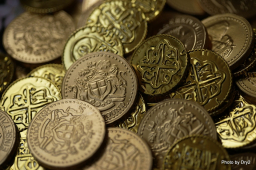Did you find an error or inaccuracy? Feel free to write us. Thank you!Tips to related online calculators
Do you want to convert time units like minutes to seconds?

## Related math problems and questions:

• Car rent 2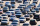The cost (c), in dollars, charged by a car rental agency is calculated using the equation c = 12 +0.29m, where m represents the distance traveled in miles. Calculate the distance traveled for the cost of $56. 37. • 7 digit numberIf 3c54d10 is divisible by 330, what is the sum of c and d? • Divisible by 5How many three-digit odd numbers divisible by 5, which are in place ten's number 3? • CommissionDaniel works at a nearby electronics store. He makes a commission of 15%, percent on everything he sells. If he sells a laptop for 293.00$ how much money does Daniel make in commission?
• Dance ensembles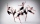4 dance ensembles were dancing at the festival. None had less than 10 and more than 20 members. All dancers from some of the two ensembles were represented in each dance. First, 31 participants were on the stage, then 32, 34, 35, 37, and 38. How many danc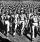Road repair took 10 days for 36 workers if they worked 9 hours a day. How many days take repair same road 37 workers if they work 8 per day?
• Five-digit numberAnna thinks of a five-digit number that is not divisible by three or four. If he increments each digit by one, it gets a five-digit number that is divisible by three. If he reduces each digit by one, he gets a five-digit number divisible by four. If it sw
• Penalty daily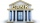John was to pay CZK 1,500. If he does not pay on time, he will be charged a penalty of 0.5 per mille of the amount owed for each day of delay. He will remember in 30 days. How much is the penalty?
• Paul earnedPaul earned 300 Kč in one hour, Václav 1/3 more than Paul. Václav worked 60 hours, which is 1/3 fewer hours than Paul worked. How many percent less earned Paul an hour than Václav? How many hours did Paul more than Václav? How much did Paul earn more than
• Parquet floor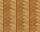Three workers laid the parquet floor for 2.301 hour. The first one would do this work alone 7 hours, the second in 6 hours. For how many hours would fulfill this work the third worker if he worked alone?
• Unknown numberSamuel wrote unknown number. Then he had add 200000 to the number and the result multiply by three. When it calculated he was surprised, because the result would have received anyway, if write digit to the end of original number. Find unknown number.
• Trees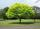Loggers wanted to seed more than 700 and less than 800 trees. If they seed in rows of 37, left them 8 trees. If they seed in rows of 43, left the 11 trees. How many trees must seed ?
• Machines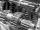In the workshop are three machines. The first is treated 250 parts for 4.7 hours, the second is treated 300 parts for 1.9 hours, and the third is treated 230 parts for 4.6 hours. How long will it take to make 3100 parts if worked all three machines at the
• Runner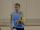Peter ran a speed of 12.4 km / h. After 45 minutes running he had a hiatus. The track is 31 km long. How many kilometers he still to run if he still running at the same speed?
• Self-counting machineThe self-counting machine works exactly like a calculator. The innkeeper wanted to add several three-digit natural numbers on his own. On the first attempt, he got the result in 2224. To check, he added these numbers again and he got 2198. Therefore, he a
• Motion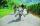Cyclist started at 9:00 from point S to point T. After 10 minutes, followed him at the same speed the second cyclist. Walker, which went from T to S, started at 9:35. After 71 minutes he met the first cyclist and after next 8 minutes the second cyclist. D
• CandyPeter had a sachet of candy. He wanted to share with his friends. If he gave them 30 candies, he would have 62 candies. If he gave them 40 candies, he would miss 8 candies. How many friends did Peter have?